Pythagorean theorem - math word problems - page 10

1. EstateEstate shaped rectangular trapezoid has bases long 34 m , 63 m and perpendicular arm 37 m. Calculate how long is its fence.
2. Euclid theoremsCalculate the sides of a right triangle if leg a = 6 cm and a section of the hypotenuse, which is located adjacent the second leg b is 5cm.
3. Square pyramidCalculate the volume of the pyramid with the side 5cm long and with a square base, side-base has angle of 60 degrees.
4. Bridge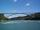The bridge arc has a span 235 m and height 3 m. Calculate the radius of the circle arc of this bridge.
5. Sphere cutsAt what distance from the center intersects sphere with radius R = 56 plane, if the cut area and area of the main sphere circle is in ratio 1/2.
6. Spherical cap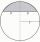What is the surface area of a spherical cap, the base diameter 20 m, height 2 m.
7. Pyramid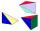Cuboid ABCDEFGH has dimensions AB 3 cm, BC 4 cm, CG 5 cm. Calculate the volume and surface area of a triangular pyramid ADEC.
8. RT and ratioA right triangle whose legs are in a ratio 6:12 has hypotenuse 68 m long. How long are its legs?
9. Trapezoid ABCD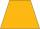Calculate the perimeter of trapezoid ABCD if we know the side c=15, b=19 which is also a height and side d=20.
10. RhombusThe rhombus with area 68 has one diagonal is longer by 6 than second one. Calculate the length of the diagonals and rhombus sides.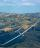Calculate how many g's (gravity accelerations) feel glider pilot when turning the horizontal circles of radius 148 m flying at 95 km/h. Centripetal acceleration is proportional to the square of the speed and inversely proportional to the radius of rotation
12. Cone containerRotary cone-shaped container has a volume 1000 cubic cm and a height 12 cm. Calculate how much metal we need for making this package.
13. Rectangle - parallelogram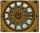It is given a rectangle that is circumscribed by a circle with a radius of 5 cm. The short side of the rectangle measures 6 cm. Calculate the perimeter of a parallelogram ABCD whose vertices are the midpoints of the sides of the rectangle.
14. AirplaneAviator sees part of the earth's surface with an area of 200,000 square kilometers. How high he flies?
15. Diamond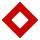Side length of diamond is 35 cm and the length of the diagonal is 56 cm. Calculate the height and length of the second diagonal.
16. Euclidean distanceCalculate the Euclidean distance between shops A, B and C, where: A 45 0.05 B 60 0.05 C 52 0.09 Wherein the first figure is the weight in grams of bread and second figure is price in USD.
17. ISO trapezoid v2bases of Isosceles trapezoid measured 12 cm and 5 cm and its perimeter is 21 cm. What is the are of a trapezoid?
18. BaseCompute base of an isosceles triangle, with the arm a=20 cm and a height above the base h=10 cm.
19. VectorsVector a has coordinates (8; 10) and vector b has coordinates (0; 17). If the vector c = b - a, what is the magnitude of the vector c?
20. TV diagonalDiagonal TV is 0.56 m long, how big the television sreen is if the aspect ratio is 16:9?

Do you have an interesting mathematical word problem that you can't solve it? Enter it, and we can try to solve it.

To this e-mail address, we will reply solution; solved examples are also published here. Please enter e-mail correctly and check whether you don't have a full mailbox.

Pythagorean theorem is the base for the right triangle calculator.